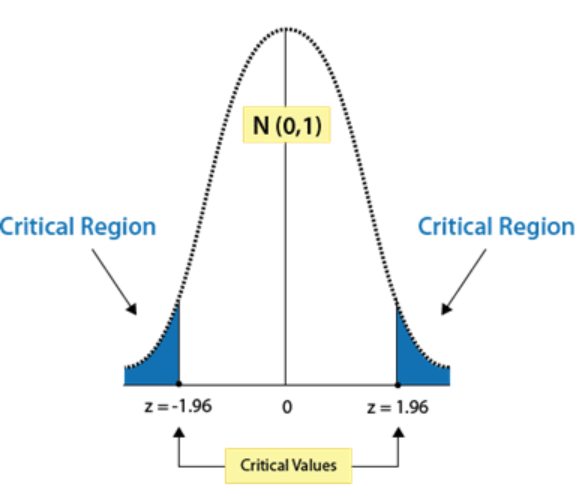# Critical Value Calculator (T & Z)

## Related Calculators

Critical value calculator is a useful tool to measure t value, z value, chi-square value as well as F and R value. Those days are gone when you needed to look in the tables and scan hundreds of values to find a corresponding value for your data.

You can use t critical value calculator to find any type of critical value. With a simple and compelling interface, our t table calculator makes it easy to perform calculations for calculating critical values for any kind of testing.

## What is a critical value?

critical value specifies the margin of error in a distribution graph when analyzing statistics. If a research statistic's absolute value is greater than the critical value, then a statistical sense denies an accepted hypothesis.## Critical value formula

The critical value formula is the standard equation for the probability.

p = 1 – α/2

Where alpha (α) represents the significance or confidence level, and p is the probability.

In this equation, the alternative hypothesis is considered as alpha. If this hypothesis is true, the null hypothesis is not accepted.

The critical value can be calculated as z score or t score if the distribution of a sampling range is usual or near to normal. You can use our f critical value calculator to find a critical value.

## How to find t critical value?

To find the critical value of t, follow the below example.

Example:

The t value is calculated by using the t distribution table. Let's assume degree of freedom as 4, alpha level of 0.025 and conduct a right-tailed test.

In the t distribution table given below, search for the degrees of freedom and its parallel alpha level. The critical value will be found at the intersection of a row and column.

 df α = 0.1 0.05 0.025 0.01 0.005 ∞ ta= 1.2816 1.6449 1.96 2.3263 2.5758 1 3.078 6.314 12.706 31.821 63.656 2 1.886 2.92 4.303 6.965 9.925 3 1.638 2.353 3.182 4.541 5.841 4 1.533 2.132 2.776 3.747 4.604 5 1.476 2.015 2.571 3.365 4.032

For the alpha level 0.025, and degrees of freedom 4, the critical value of t will be 2.776.

T critical value = 2.776.

Moreover, you can use our t value calculator to find the t value at one click.

## How to find z critical value?

Example:

We will assume significance level (α) of 0.03 to find the z critical value for two tailed test.

Step 1: Divide the significance level by 2.

α/2 = 0.03/2

α/2 = 0.015

Step 2: Calculate (1 - α/2).

1 - α/2 = 1 – 0.015

1 - α/2 = 0.985

Step 3: Look for 0.985 in z table. Add the corresponding value of first row and most left (first) column.

2.1 + 0.07 = 2.17

Z value = ± 2.17

Use z critical value calculator if you are not into prolonged calculations.

## What is Chi-Square Value?

One way to show a relationship between two categorical variables is a Chi-square statistic.

The chi-square statistic compares two different variables to determine whether they are related. The numbers are determined by looking at the Chi-square table. Our chi square critical value calculator above eliminates the need to look for values in the tables.

#### Need Custom Calculator?

With Over Online Tools, eCalculator.co Helping Millions of Students, Teachers, Businessmen & Nutritionists Every Month.